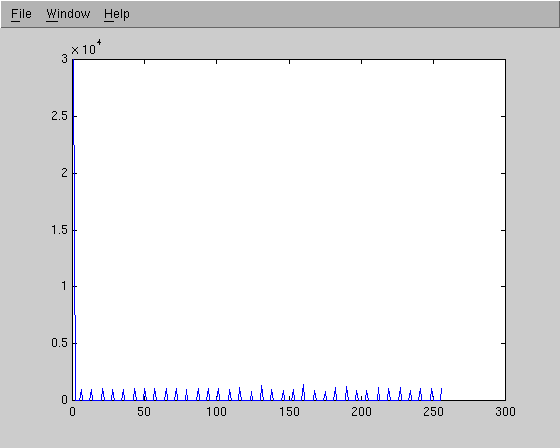Image Processing Lab
EC480/PH437
Lab 3
Bryan Evenson and Ted Gould

For this lab, we were to play with the histogram features of MATLAB with a moon image and an additional image of our choice.  We started out with the image shown below.

Our ImageFirst, we took the histogram of the image, which is shown below.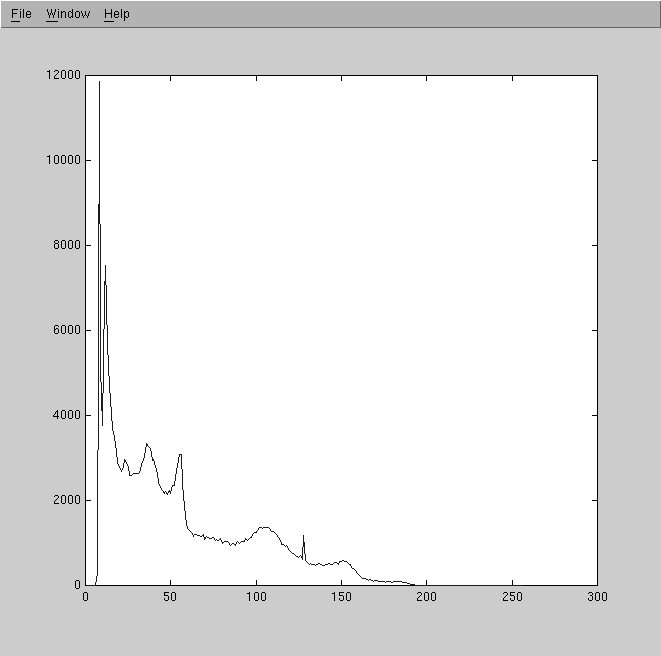We then performed a histogram stretch of this image.MATLAB code:
imshow(imadjust(IM, [0 0.5], [0 1], 1);

Histogram of stretched image: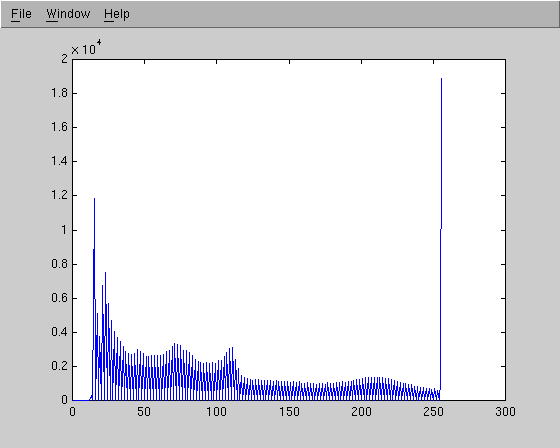We then inverted the image using imadjust()MATLAB code:
imshow(imadjust(IM, [0 1], [1 0], 1));

Here's the histogram of the image: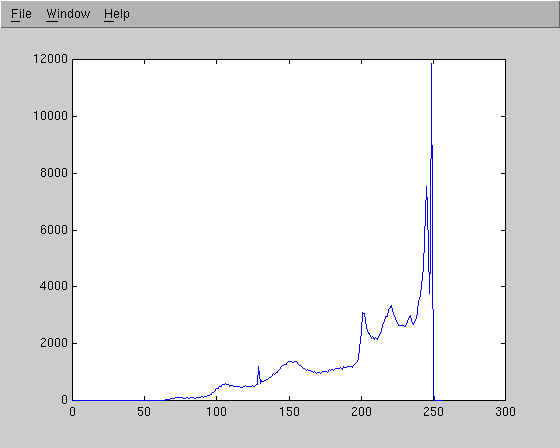Next, we changed the gamma parameter of the imadjust() function.  Below are the results of using gamma values of 0.2, 0.5 and 2.0 respectively.Moon image

We started playing with this image like the last one, by first taking the histogram of the image.

Original image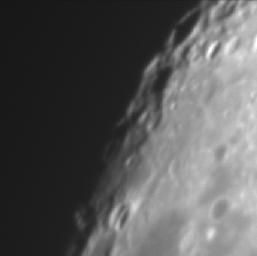Histogram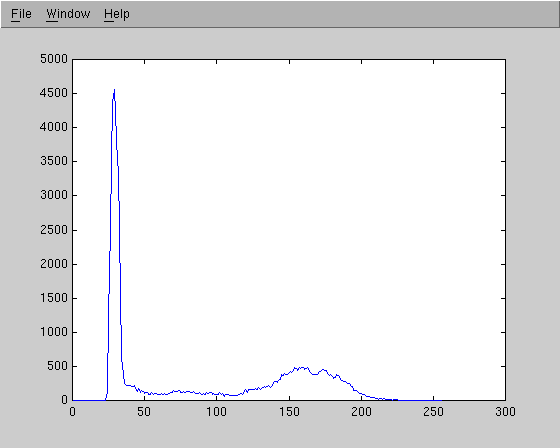Then, we used histeq() to perform a histogram equalization on the image.

Histogram equalized image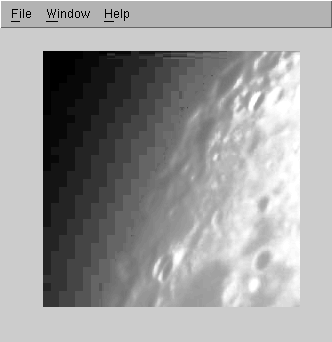Histogram of previous image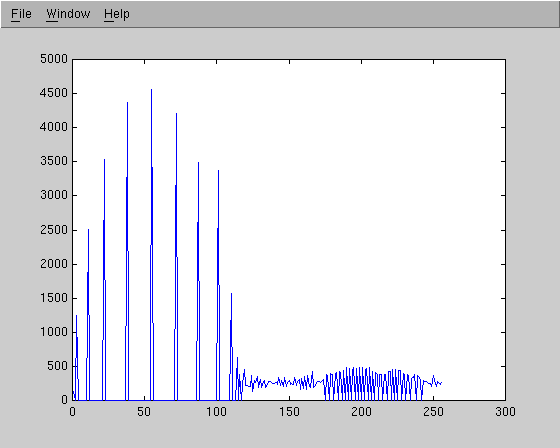The main problem with histogram equalization on this image is that it emphasizes the wrong set of values.  It emphasizes the big block of dark values by spreading them out.  In this image the light values are more important to the detail, and in this case they were deemphasized.
To fix this problem, we took all the pixels that had less than 115 in the histogram and gave them the value of 0.  We then performed a histogram stretch of the rest of the values in the image.  Here's the results:

Good looking image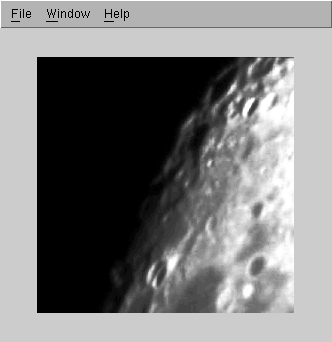MATLAB code:
imshow(imadjust(histeq(IM), [.45 1], [0 1], 1))

Histogram of good image